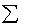previous lesson

Kruskal - Wallis H

INFERENCE: one nominal and one ordinal variable

A. Kruskal-Wallis analysis of variance (H):
1. Rank order all ordinal scores in all nominal categories.
2. Calculate the sum of ranks for each nominal category.
3. Substitute into the formula:

H = 12/[N(N + 1)] [Rj2 / nj ] - 3(N + 1)

Where: N = total in sample
nj = number in a nominal category
k = number of nominal categories
Rj = sum of ranks for a nominal category

4. Look up p-value of H in the Chi Square Significant Probability Value using the degrees of freedom formula: k - Assumptions:

• continuous distribution underlying the ordinal variables- a continuous variable is one whose unit of measure can be divided infinitely; ordinal continuous data resembles interval/ratio data in its wide possibilities of response; instead of just five or six (i.e. scores, scales, etc.)
• five or more cases in each nominal category.

Example: Data without ties on the ordinal variable

 Political Interest Scores South North East West 35 36 40 41 34 31 30 28 22 23 26 27 21 20 17 14 8 9 11 13

Q: Can this be generalized to the whole population?
A: Variables: region of residence (nominal) and political interest scores (ordinal).
Check to be sure assumptions are met before proceeding. (Okay in this example)

 South Rank North Rank East Rank West Rank 35 17 36 18 40 19 41 20 34 16 31 15 30 14 28 13 22 9 23 10 26 11 27 12 21 8 20 7 17 6 14 5 8 1 9 2 11 3 13 4 51=Rs 52=Rn 53=Re 54=Rw

*Note: When ranking the scores, ranking can either be from high to low or low to high.

RS = sum of ranks for south. nS = number in south = 5
RN = " " " " north. nN = " " "north = 5
RE = " " " " east. nE = " " " east = 5
RW = " " " " west. nW = " " " west = 5 and N = 20

H = 12/[20(20+1)] [(51)2/5 + (52)2/5+ (53)2/5 + (54)2/5] - 3(20 + 1) =

H = .0285714 [ 2206 ] - 63**Note: Keep as much accuracy as possible for this statistic.

H = 63.028508 - 63

H =.03 (At the end, round answer to 2 decimal places.)

Look this up on Chi Square table: d.f. = k - 1 = 4 - 1 = 3

In this case, p value > .05 so one cannot generalize the difference to the whole population.

Example: Data with ties on the ordinal variable:

 Abortion Attitude Scores Hi MedHi MedLo Lo Experimental group #1 5 1 0 0 6 Experimental group #2 0 1 1 5 7 Control group 0 3 4 0 7 5 5 5 5 20

Q: Can this be generalized to the whole population?
A: Put data in a table with nominal variable on left side and ordinal variable on top. Use same formula and same procedure.

Dealing with rank scores in data with ties: scores from an exam:

Score Rank

99 -----1

97 -----2.5 If these were ranked #2 and #3 it wouldn't

97 -----2.5 be fair since they have the same score, so

93 -----4 split the difference.

92 -----5

90 -----7 Same for here: Instead of numbers 6,7 and 8,

90 -----7 split the difference and gave it to each.

90 -----7

89 -----9

In the example above, take the column totals and make up a table:

 Abortion Attitude Scale Hi MedHi MedLo Lo Column totals 5 5 5 5 Rank range 1-5 6-10 11-15 16-20 Median range 2 8 13 18

R exp #1 = 5(3) + 1(8) = 23; n exp #1 = 6

R exp #2 = 1(8) + 1(13) + 5(18) = 111; n exp #2 = 7

R control = 3(8) + 4(13) = 76; n control = 7

H = 12/[20(20+1)] [(23)2/6 + (111)2/7 + (76)2/7] - 3(20+1) = .0285714 [ 2673.45 ] - 63

H = 76.384209 - 63 = 13.38

Look this value up on the Chi Square table, (d.f.= 3 - 1 = 2) and find that H is greater than p < .01 so one can generalize to the entire population.